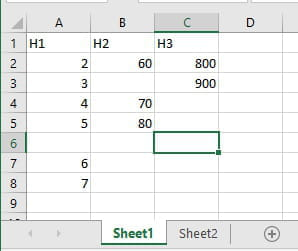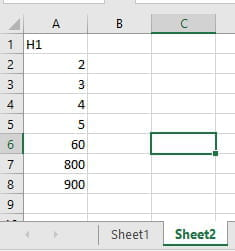# Return Seq Values Until Blank value then return different set of seq values

-
Posts
2818
Registration date
Sunday September 12, 2010
Status
Moderator
Last seen
October 18, 2021
-
Hello,
What is a formula to return a specific set of values in sequential cells until the reference sequence returns a blank value then start returning values from a different sequence?

Example:

if A2 is not blank, return value in A2 then sequential as A3, A4 etc. else return value starting in B1, then sequential as B2, B3 etc…

Posts
2818
Registration date
Sunday September 12, 2010
Status
Moderator
Last seen
October 18, 2021
486
Hi Lorieb,

When your source sheet looks like this:and you want your destination sheet to look like this:Then this is the code to use:
```Sub RunMe()
Dim r, c As Long
r = 2
c = 1
Sheets("Sheet1").Select
Do
Sheets("Sheet2").Range("A" & Rows.Count).End(xlUp).Offset(1, 0).Value = Cells(r, c).Value
r = r + 1
If Cells(r, c).Value = vbNullString Then
r = 2
c = c + 1
End If
Loop Until Cells(r, c) = vbNullString
End Sub```

Best regards,
Trowa